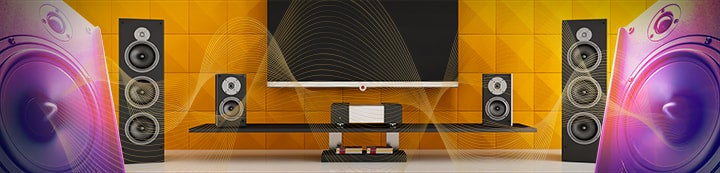1 - 7 of 7 Posts

#### rentangz

·
##### Registered
Joined
·
33 Posts
Discussion Starter · ·
Looking on the logarithmic room response graph, why is it that the spacing/calibration of the scale not uniform even if the frequency difference is uniform and the spacing becomes narrower at certain frequency band?Is it because there are particular frequency band of interest such that it is stretched and wider and narrower for other band that of lesser interest?

#### SturmMD

·
##### Banned
Joined
·
165 Posts
The varying spacing is because the ear hears things on a nonlinear frequency scale. For example, an octave is a doubling in frequency and music scales follow this pattern too. So, for the frequency sweep to sound linear it must sweep logarithmically, i.e. on a power scale not linear scale.

The logarithmic graph is a better way of looking at wide frequency ranges. Imagine if you had a normal graph from 10 to 20,000. The 10 to 100Hz part would be small.

Also, if you take a ruler and physically measure the distance between 10Hz and 20Hz on the graph, you will see that that distance is equal to any other distance between two frequencies which are a multiple of 2 of each other. For example, 40Hz and 80Hz.

#### robbo266317

·
##### Registered
Joined
·
5,772 Posts
As Sturm said, our hearing is non-linear.
Also this is why we measure sound level in decibels - another logarithmic scale - because that is how we "hear" it.
3dB is double in power, however we perceive 10dB to be twice as loud!

#### rentangz

·
##### Registered
Joined
·
33 Posts
Discussion Starter · ·
Thanks for the explanation guys...I am aware that we hear on a logarithmic scale but I am just curious how is the graph plotted that way whereby some band are narrow and some wide..I mean how did we determine the physical spacing in plotting or making the graph?Is the graduation a standard or varies depending on the frequency band of interest?

#### SturmMD

·
##### Banned
Joined
·
165 Posts
The spacing is determined by the logarithm function. Take the logarithm of a frequency and plot the answer on a new linear scale. For example (base 10) log(10)=1, log(20)=1.301, log(40)=1.602, log(100)=2.0. So in the plot 10 and 100Hz are separated by 1 unit, 20 and 40Hz by 0.301 units, etc.

#### brucek

·
##### Registered
Joined
·
7,514 Posts
how did we determine the physical spacing in plotting or making the graph?
That's debatable. Some say it was Joost Bürgi of Switzerland who discovered logarithms in 1610, but didn't write about it until after John Napier of Scotland published the first book on it in 1614.

Did you never work with log graphs in school?

brucek

#### rentangz

·
##### Registered
Joined
·
33 Posts
Discussion Starter · ·
Thanks for your insights brucek and forgive me if I may sound elementary on this logarithmic scaling maybe I was not clear with my inquiry but I think SturmMD has illustrated the relation between the actual linear spacing on paper and plot on how to get the spacing based on the calculation he gave to get the units spacing..this will explain the varying spacing on the graph in relation to the frequency..I am familiar with this log graph in my electrical engineering years before but was not able to dig back on my notes on this log plotting..hehheh..anyway thanks very much...:heehee:

1 - 7 of 7 Posts